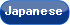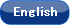Please use this identifier to cite or link to this item: `http://hdl.handle.net/10119/4915`

 Title: Parallel Approximation Algorithms for Maximum Weighted Matching in General Graphs Authors: Uehara, RyuheiChen, Zhi-Zhong Keywords: Graph algorithmmaximum weighted matchingapproximation algorithmparallel algorithm Issue Date: 2000 Publisher: Springer Magazine name: Lecture Notes in Computer Science Volume: 1872 Start page: 84 End page: 98 DOI: 10.1007/3-540-44929-9_7 Abstract: The problem of computing a matching of maximum weight in a given edge-weighted graph is not known to be P-hard or in RNC. This paper presents four parallel approximation algorithms for this problem. The first is an RNC-approximation scheme, i.e., an RNC algorithm that computes a matching of weight at least 1 - ε times the maximum for any given constant ε > 0. The second one is an NC approximation algorithm achieving an approximation ratio of 1/(2+ε) for any fixed ε > 0. The third and fourth algorithms only need to know the total order of weights, so they are useful when the edge weights require a large amount of memories to represent. The third one is an NC approximation algorithm that finds a matching of weight at least 2/(3Δ+2) times the maximum, where Δ is the maximum degree of the graph. The fourth one is an RNC algorithm that finds a matching of weight at least 1/(2Δ+4) times the maximum on average, and runs in Ο(log Δ) time, not depending on the size of the graph. Rights: This is the author-created version of Springer, Ryuhei Uehara and Zhi-Zhong Chen, Lecture Notes in Computer Science, 1872, 2000, 84-98. The original publication is available at www.springerlink.com, http://dx.doi.org/10.1007/3-540-44929-9_7 URI: http://hdl.handle.net/10119/4915 Material Type: author Appears in Collections: b10-1. 雑誌掲載論文 (Journal Articles)

Files in This Item:

File Description SizeFormat